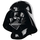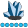## ODS PDF Layout Gridded

Hello!

I am trying to put multiple sgplots on one page using ods pdf and ods layout gridded format. This has been very temperamental but I have it almost all the way there but it seems that when I include the legend with my graph in the second column then the next graph in the first column will not print in the pdf. Why would this be?? In the code below I exemplified it by having the graph in the second column first row with no legend and the graph in the second column second row include a legend.

libname KPI '...';

data kpi.activeQOF ;

set kpi.activeQOF;

run;

ODS listing close;

Option orientation=portrait;

goptions reset=all

ftext="swissb";

ods PDF file="....." startpage=never ;

ods layout Start height=11in width=8in columns=2 rows=3

column_gutter=.5in row_gutter=.2in row_heights=(3.5in 3.5in 3.5in);

ods region row=1 column=1;

proc sgplot data=kpi.activeQOF ;

title2 'PLOT #1';

series y=HHSIZE1Mem x=Week ;

series y=HHSIZE2Mem x=Week ;

series y='hhsize3/4mem'n x=Week ;

series y='HHSIZE-5+Mem'n x=Week ;

run;quit;

ods region row=1 column=2;

proc sgplot data=kpi.activeQOF noautolegend;

title2 'PLOT #2';

series y='INCOME-<\$25k'n x=Week;

series y='INCOME-\$25-35k'n x=Week;

series y='INCOME-\$35-50k'n x=Week;

series y='INCOME-\$50-69k'n x=Week;

series y='INCOME-\$70-100k'n x=Week;

series y='INCOME-\$100k+'n x=Week;

run;quit;

ods region row=2 column=1;

proc sgplot data=kpi.activeQOF ;

title2 'PLOT #3';

series y='HHAGE-HH<35'n x=Week ;

series y='HHAGE-HH35-54'n x=Week ;

series y='hhage-55+'n x=Week ;

run;quit;

ods region row=2 column=2;

proc sgplot data=kpi.activeQOF ;

title2 'PLOT #4';

series y='HHRACE-White'n x=Week ;

series y='HHRACE-Black'n x=Week ;

series y='HHRACE-OTHER'n x=Week ; ;

run;quit;

ods region row=3 column=1;

proc sgplot data=kpi.activeQOF;

title2 'PLOT #4';

series y=HHSIZE1Mem x=Week ;

run;quit;

ods region row=3 column=2;

proc sgplot data=kpi.activeQOF;

title2 'PLOT #4';

series y=HHSIZE1Mem x=Week ;

run;

quit;

ods layout end;

ods pdf startpage=now;

ods layout start width=8in height=11in columns=2 rows=3

column_gutter=.5in row_gutter=.2in row_heights=(3.5in 3.5in 3.5in);

ods region row=1 column=1;

proc sgplot data=kpi.activeQOF;

title2 'PLOT #4';

series y=HHSIZE1Mem x=Week ;

run;quit;

ods region row=1 column=2;

proc sgplot data=kpi.activeQOF;

title2 'PLOT #4';

series y=HHSIZE1Mem x=Week ;

run;quit;

ods region row=2 column=1;

proc sgplot data=kpi.activeQOF;

title2 'PLOT #4';

series y=HHSIZE1Mem x=Week ;

run;quit;

ods region row=2 column=2;

proc sgplot data=kpi.activeQOF;

title2 'PLOT #4';

series y=HHSIZE1Mem x=Week ;

run;quit;

ods region row=3 column=1;

proc sgplot data=kpi.activeQOF;

title2 'PLOT #3';

series y=HHSIZE1Mem x=Week ;

run;quit;

ods region row=3 column=2;

proc sgplot data=kpi.activeQOF;

title2 'PLOT #4';

series y=HHSIZE1Mem x=Week ;

run;

quit;

ods layout end;

ods PDF close;DartRodrigo
Lapis Lazuli | Level 10

## Re: ODS PDF Layout Gridded

Hi mate,

I've made a seach and found out that you used some example of a PDF website that has the same attributes,

But in order, i've found another option to do this with proc greplay that i concern, it is possible to put multiple

graphs side by side and so on. Or if you are using SAS Enterprise Guide you can create a Point-and-click report

and export to PDF.

Hope this helpsAtt

Discussion stats